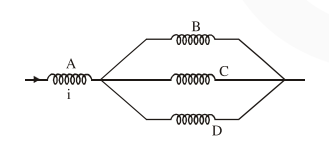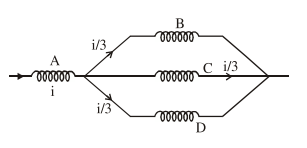# Four identical long solenoids A,B,C and D are`
Question:

Four identical long solenoids $\mathrm{A}, \mathrm{B}, \mathrm{C}$ and $\mathrm{D}$ are connected to each other as shown in the figure. If the magnetic field at the center of $\mathrm{A}$ is $3 \mathrm{~T}$, the field at the center of $\mathrm{C}$ would be : (Assume that the magnetic field is confined with in the volume of respective solenoid).1. $12 \mathrm{~T}$

2. $6 \mathrm{~T}$

3. $9 \mathrm{~T}$

4. $1 \mathrm{~T}$

Correct Option: , 4

Solution:$\phi \propto \mathrm{i}$

$\Rightarrow B \propto i$

so, field at centre of $\mathrm{C}=\frac{3}{3}=1 \mathrm{~T}$